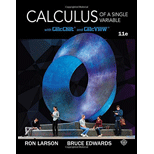Chapter 8.4, Problem 28E### Calculus of a Single Variable

11th Edition
Ron Larson + 1 other
ISBN: 9781337275361

#### Solutions

Chapter
Section### Calculus of a Single Variable

11th Edition
Ron Larson + 1 other
ISBN: 9781337275361
Textbook Problem

# Finding an Indefinite Integral In Exercises 19-32, find the indefinite integral. ∫ 1 − x x   d x

To determine

To calculate: The solution of the indefinite integral 1xxdx.

Explanation

Given:

The indefinite integral is 1xxdx.

Formula used:

The trigonometric identity, cos2θ=1sin2θ and cos2θ=1+cos2θ2.

The integral identity cosθdθ=sinθ+C.

Calculation:

Because 12x2 is of the form a2x2. So, make use of the trigonometric solution, x=sinθ to solve it as shown in figure.

Differentiate the above equation is;

12xdx=cosθdθ

Therefore;

1xxdx=21sin2θcosθdθ=2cos2θdθ=1+cos2θdθ=(θ+sin2θ2

### Still sussing out bartleby?

Check out a sample textbook solution.

See a sample solution

#### The Solution to Your Study Problems

Bartleby provides explanations to thousands of textbook problems written by our experts, many with advanced degrees!

Get Started

## Additional Math Solutions

#### Evaluate the integral. 7. 0/2cos2d

Single Variable Calculus: Early Transcendentals

#### In Exercises 63-68, use the graph of the function f to determine limxf(x) and limxf(x) 64.

Applied Calculus for the Managerial, Life, and Social Sciences: A Brief Approach

#### Convert the expressions in Exercises 6584 to power form. 3x25472x3

Finite Mathematics and Applied Calculus (MindTap Course List)